##### HTML5 and CSS3 All-in-One For DummiesThe birthday value is stored in the hero table in SQL, but what you really want to know as an HTML5 programmer is the hero's age. It's very common to have a date stored in a database. You often need to calculate the time from that date to the current date in years, or perhaps in years and months. Functions can help you do these calculations.

## Begin by looking at a simple function that tells you the current date and time.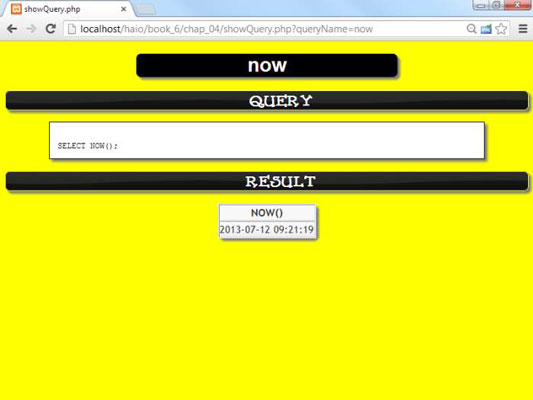The current date and time by themselves aren’t that important, but you can combine this information with other functions to do some very interesting things.

## How to use DATEDIFF to determine age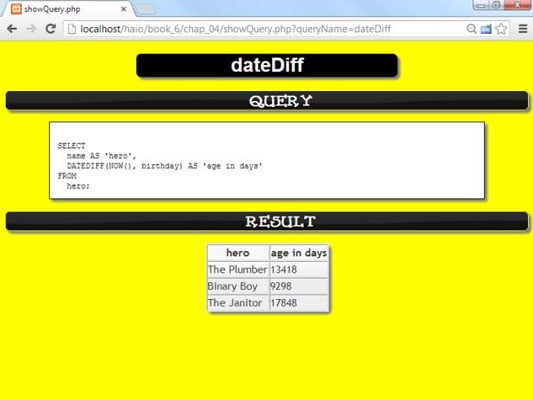The NOW() function is very handy when you combine it with the DATEDIFF() function.

This query calculates the difference between the current date, NOW(), and each hero’s birthday. The DATEDIFF()function works by converting both dates into integers. It can then subtract the two integers, giving you the result in number of days.

You normally name the fields you calculate because otherwise, the formula used to calculate the results becomes the virtual field’s name. The user doesn’t care about the formula, so use the AS feature to give the virtual field a more useful name.

## How to add a calculation to get years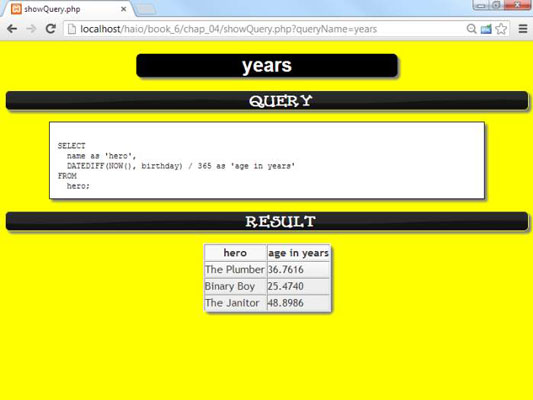Of course, most people don’t think about age in terms of days. Age (unless you’re talking about fruit flies or something) is typically measured in years. One simple solution is to divide the age in days by 365 (the number of days in a year).

This code uses a mathematical operator. You can use most of the math operators in queries to do quick conversions. Now, the age is specified in years, but the decimal part is a bit odd. Normally, you either go with entire year measurements or work with months, weeks, and days.

## How to convert the days integer into a date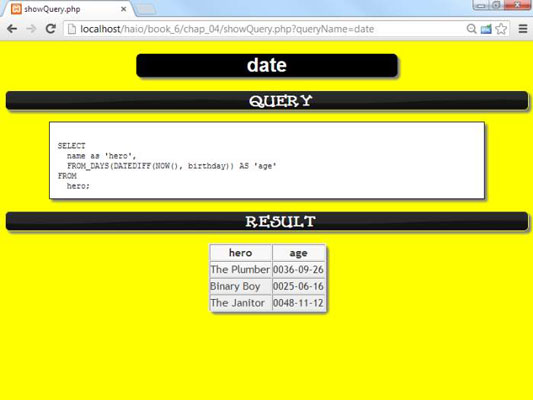The YEAR() function extracts only the years from a date, and the MONTH() function pulls out the months, but both these functions require a date value. The DATEDIFF()function creates an integer. Somehow, you need to convert the integer value produced by DATEDIFF() back into a date value.

This query takes the value and converts it back to a date. The actual date is useful, but it has some strange formatting. If you look carefully at the dates, you’ll see that they have the age of each hero, but it’s coded as if it were a particular date in the ancient world.

## How to use YEAR() and MONTH() to get readable values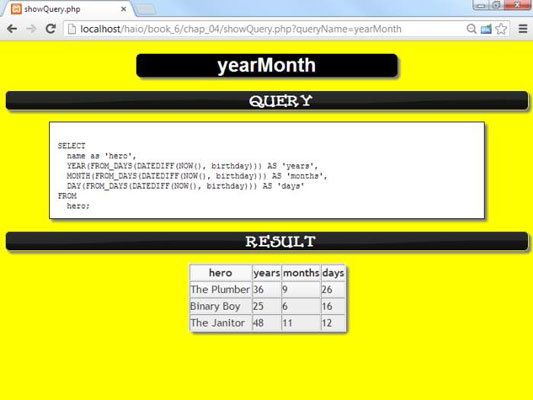After you’ve determined the age in days, you can use the YEAR() and MONTH() functions to pull out the hero’s age in a more readable way.

The query is beginning to look complex, but it’s producing some really nice output. Still, it’s kind of awkward to have separate fields for year, month, and day.

## Concatenating to make one field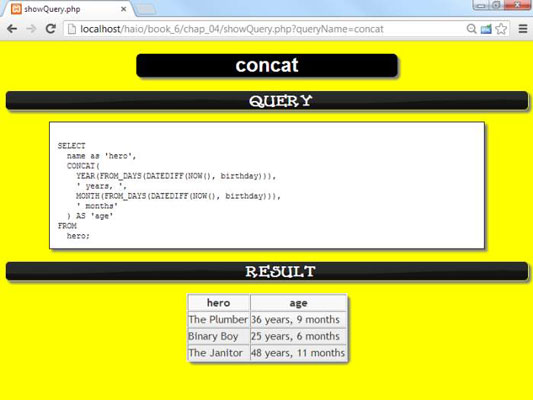If you have year, month, and day values, it would be nice to combine some of this information to get a custom field.

This query uses the CONCAT() function to combine calculations and literal values to make exactly the output the user is expecting. Even though the birthday is the stored value, the output can be the age.